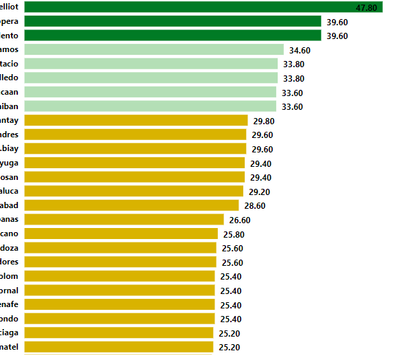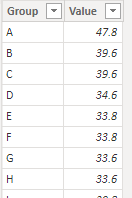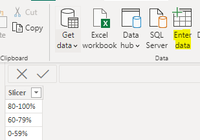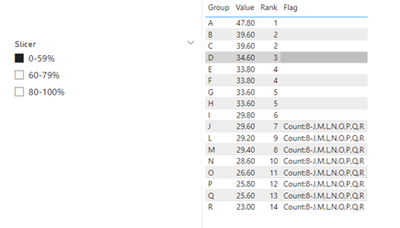cancel
Showing results for
Did you mean:Helper V

## Counting top 80-100% in rank

Hello, currently doing a ranking for agents a scorecard to be exact and their score is on whole number not in percent. The thing is i want to count the agent that fall under 80-100%, 60-79%, and 0-59% and put it in a Card to show in my Executive Summary. My roll up score or their final score is in calculated column. Kindly see the photo below for reference. Thanks in advance!5 REPLIES 5Solution Sage

Though not entirely relevant, this video might help you with the concept:Community Support

Hi  @xRTP ,

I created some data:Here are the steps you can follow：

1. Enter data – create a table.2. Create measure.

``````Rank =
RANKX(
ALL('Table'),CALCULATE(SUM('Table'[Value])),,DESC,Dense)``````
``````Flag =
VAR _count =
CALCULATE ( DISTINCTCOUNT ( 'Table'[Group] ), ALL ( 'Table' ) )
VAR _08 = _count * ( 1 - 0.8 ) // 3
VAR _06 = _count * ( 1 - 0.6 ) // 6.8
VAR _079 = _count * ( 1 - 0.79 ) //4
VAR _059 = _count * ( 1 - 0.59 ) //6.97
RETURN
SWITCH (
TRUE (),
MAX ( 'Slicer_Table'[Slicer] ) = "80-100%"
&& [Rank] <= _08,
"Count" & ":"
& COUNTX ( FILTER ( ALL ( 'Table' ), 'Table'[Rank] <= _08 ), [Group] ) & "-"
& CONCATENATEX ( FILTER ( ALL ( 'Table' ), 'Table'[Rank] <= _08 ), [Group], "," ),
MAX ( 'Slicer_Table'[Slicer] ) = "60-79%"
&& [Rank] >= _079
&& [Rank] <= _06,
"Count" & ":"
& COUNTX (
FILTER ( ALL ( 'Table' ), 'Table'[Rank] >= _079 && 'Table'[Rank] <= _06 ),
[Group]
) & "-"
& CONCATENATEX (
FILTER ( ALL ( 'Table' ), [Rank] >= _079 && [Rank] <= _06 ),
[Group],
","
),
MAX ( 'Slicer_Table'[Slicer] ) = "0-59%"
&& [Rank] >= _059,
"Count" & ":"
& COUNTX ( FILTER ( ALL ( 'Table' ), 'Table'[Rank] >= _059 ), [Group] ) & "-"
& CONCATENATEX ( FILTER ( ALL ( 'Table' ), 'Table'[Rank] >= _059 ), [Group], "," )
)
``````

3. Result:Best Regards,

Liu Yang

If this post helps, then please consider Accept it as the solution to help the other members find it more quicklyHelper V

Hello, this is awesome.

Im trying to add a new filter on the measure but i only get error, I have a calculated column named "Met/Not Met" and flag agents there if their score has met or not, so before the ranking i should filter agents who was flagged as "Met". Is it possible?Super User

If I took the time to answer your question and I came up with a solution, please mark my post as a solution and /or give kudos freely for the effort 🙂 Thank you!

Proud to be a Super User!Helper V

Whats your email? I'll email it to you. Thanks!